# XMM-Newton Users Handbook

### 3.5.5 OM sensitivity and detection limits

Both, Fig. 106 and Table 20, provide the expected count rate of the OM, in counts per second, for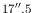mag stars of various types, with the different filters introduced above. These numbers were obtained under the assumption of a perfect detector, i.e., free of deadtime and coincidence loss, and without time sensitivity degradation, and with an aperture radius of(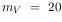) for optical (UV) filters.

 Filter B0 A0 F0 G0 G2 K0 M0 WD V 1555 1510 1473 1473 1495 1452 1392 1412 B 6190 5272 3805 3010 2577 2289 1337 4454 U 8533 2216 1486 1119 966 444 178 4667 UVW1 5647 958 366 218 182 29.1 17.4 3093 UVM2 2276 316 39.3 8.69 5.54 0.436 0.032 1174 UVW2 950 126 11.1 2.06 1.19 0.112 0.069 561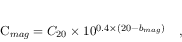The numbers listed in Table 20 can be used to calculate the perfect detector count rates of stars of given magnitude by the formula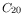(3)

where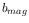is the count rate of anmag star of the same spectral type as the target, andand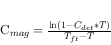are theand the count rate of the target of interest, respectively.

However, OM deadtime and coincidence losses must be taken into account, for instance using SAS. For the 20th magnitude stars in Fig. 106 and Table 20, OM coincidence losses are negligible. Losses become significant for a point source at a count rate of about 10 counts s(about 10% coincidence) for a full frame exposure.

The correction is approximated by the following formula:(4)

whereis the count rate of incident photons from equation (3),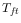is the actual measured count rate,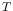is the CCD frametime in units of seconds andthe frametransfer time in the same units (0.1740 ms). The frametime is a function of the OM science window configuration, as described in § 3.5.2.

An optimum aperture radius ofwas found to be the radius at which the loss correction formula is self consistent. At this radius the accuracy of the coincidence loss correction is at a level of 5% between different frametimes.

The validity range of the coincidence loss correction can be obtained from eq. 4 as a function of the frametime, whose maximum value (11.04 ms) occurs for full frame exposures, high or low resolution, where the whole detector is used. In windowed exposures the frametime varies from about 5 to 10 ms. For this reason the maximum measured count rates that can be reliably corrected are in the range of a few hundreds counts per second. Higher rates (1000 cts/s) may not damage the detector, but are scientifically useless.

We give in Table 21 coincidence loss corrected rates for limit high measured rates. The table gives the maximum measurable rate for each given value of frametime (1 count per frametime) and the corresponding corrected values for the maximum rate minus 0.01 and 0.001 c/s. For these high rates we obtain correction factors of 10, and very small differences in count rate produce variations in the corrected rates of more than 20%.

 Frametime Maximum Corrected rate Corrected rate measured rate max-0.01 max-0.001 (ms) (c/s) (c/s) (c/s) 5 200 2052 2531 7 143 1402 1739 9 111 1056 1317 11 91 842 1054

The coincidence loss correction implemented in SAS (from version 14) sets a validity limit at a rate of 0.97 counts per frametime (source+background). Within this limit, the correction error is less than 2%. This corresponds to about 700 and 300 source+background corrected counts for frametimes of 5 and 11 ms, respectively. For values higher than 0.97, the source counts are not corrected by SAS, and therefore, the conversion to magnitudes and fluxes are not applied. The produced source list contains only the measured count rate and position for these sources.

In Fig. 107, a comparison between formula (4) and the inflight instrument performance is shown.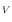In Table 22, an estimate of the limiting magnitudes that can be detected with OM in an integration time of 1000 s at the 5-sigma confidence level is provided. They have been obtained from simulations, taking into account the whole aperture (radius for optical filters andfor UV) on which the OM photometric calibration is based. If we consider that detections can be obtained in the very few pixels in the core of the PSF, since the total background is then lower, the limit would be about one magnitude fainter.

 Filter Spectral type B0 A0 G0 K0 WD V 19.8 19.8 19.7 19.7 19.7 B 21.0 20.8 20.2 19.9 20.6 U 21.8 20.4 19.6 18.6 21.2 UVW1 21.1 19.2 17.6 15.4 20.5
Notes to Table 22:
1) Numbers obtained adopting as background a zodiacal light level of 100 S10 units, where S10 is the brightness of one m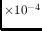=10 mag solar type star per square degree. All magnitudes are in V filter.

Table 23 presents the limiting magnitudes derived from the photometric analysis of one of the OM calibration fields (HD5980, also observed from the ground). This can be considered as an extreme case because of the crowdedness of the field which increases the observed background by overlap of the PSF wings. This is why the obtained limits are brighter than the simulations of Table 22.

 Filter Spectral type range B0/A0 A1/G3 G4/M8 V 19.5 19.4 19.4 B 20.6 19.8 19.3 U 20.2 19.4 18.6
Notes to Table 23:
1) Based on OM observations of HD5980 field, obtained with V, B and U filters. Magnitudes are standard V.

A detailed study of the background sources in OM can be found in:
http://www.cosmos.esa.int/documents/332006/623312/MSSLbckgd_v6.pdf
http://www.cosmos.esa.int/documents/332006/623312/OMbackg05.pdf.
The expected levels of different external background radiation processes in the optical/UV are tabulated in Table 24. The background count rate in the OM is dominated by the zodiacal light in the optical. In the far UV the intrinsic detector background becomes important. Images are regularly taken with the blocked filter and no LED illumination to measure the detector dark counts. The mean OM dark count rate is 4.0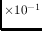counts spixel. The variation across the detector is9% , with a mainly radial dependence, being highest in an annulus of about 8' radius and lowest at the centre. Variations as a function of time are7% , without any apparent trend. However, if a very bright star is in the field of view, the dark rate can be up to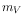60% higher than normal, despite the use of the Blocked filter.

 Background source Occurrence Count rate range Diffuse Galactic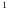all directions 2.14-7.527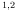Zodiacal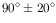longitude1.69-5.611Average dark count rate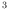all directions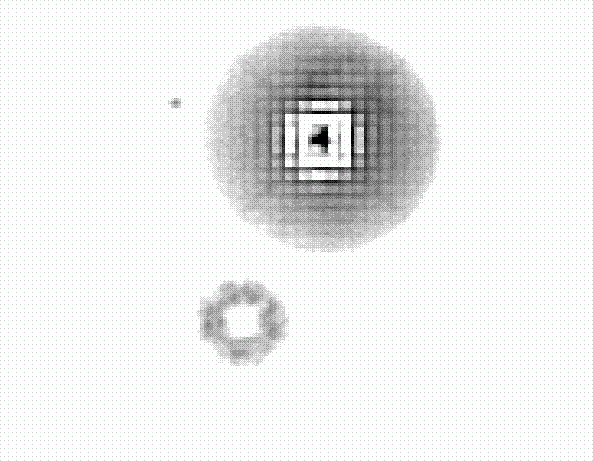Notes to Table 24:
1) In units of [counts sarcsec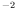].
2) In differential ecliptic coordinates, i.e., the angle between the Sun and the pointing position of XMM-Newton. Maximum is for l,b = 70,0 in the white filter, minimum is for l,b = 110,70 in the UVW2 filter.
3) In units of [counts spixel]

Artifacts can appear in the XMM-Newton OM images due to light being scattered within the detector. These have two causes: internal reflection of light within the detector window and reflection of the off-axis starlight and background light from part of the detector housing.

The first of these causes a faint, out of focus ghost image of a bright star, displaced in the radial direction away from the primary image (see Fig. 108).The second effect is due to light reflecting off a chamfer in the detector window housing. Bright stars that happen to fall in a narrow annulusto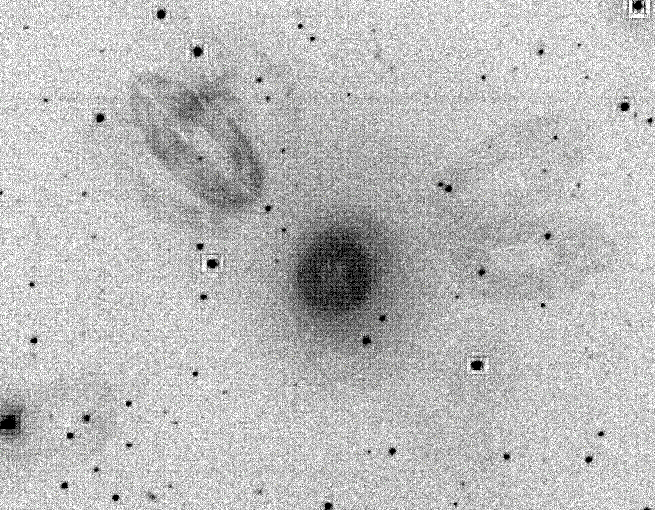off axis shine on the reflective ring and form extended loops of emission radiating from the centre of the detector (see Fig. 109).Similarly, there is an enhanced “ring” of emission near the centre of the detector due to diffuse background light falling on the ring. These features are less prominent when using UV filters, due to the lower reflectivity of UV light.

Figure 110 shows the low sensitivity patch produced by an accidental observation of Jupiter in July 2017. This figure shows also the "ring" mentioned before. The low sensitivity patch is oval with a size aroundpix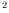, equivalent to 1.4 square arcmin approximately. The whole field is 17 arcmin in diameter.European Space Agency - XMM-Newton Science Operations Centre